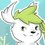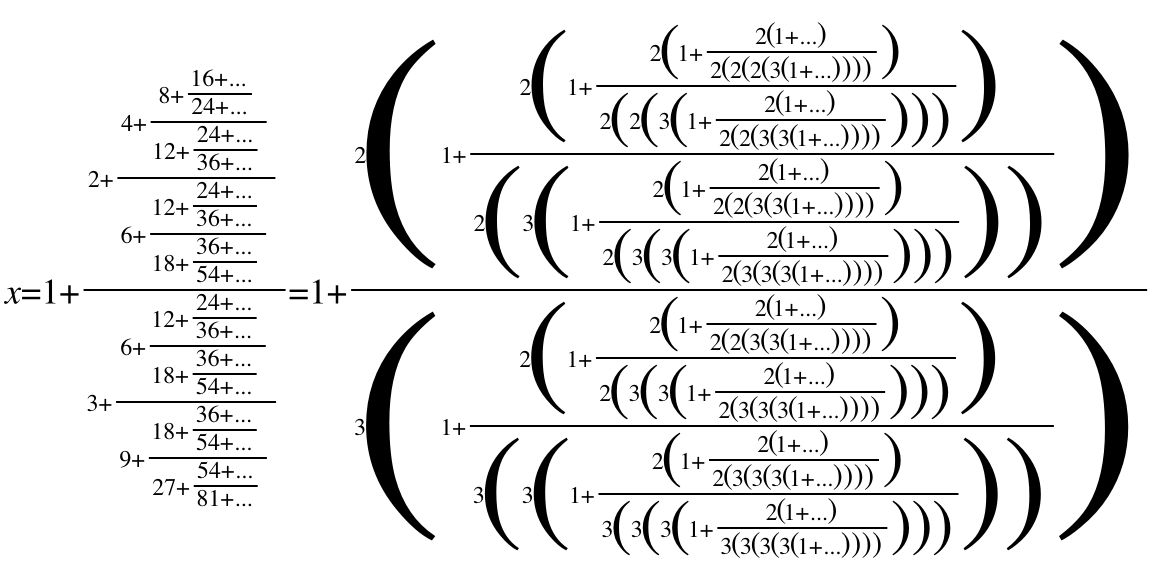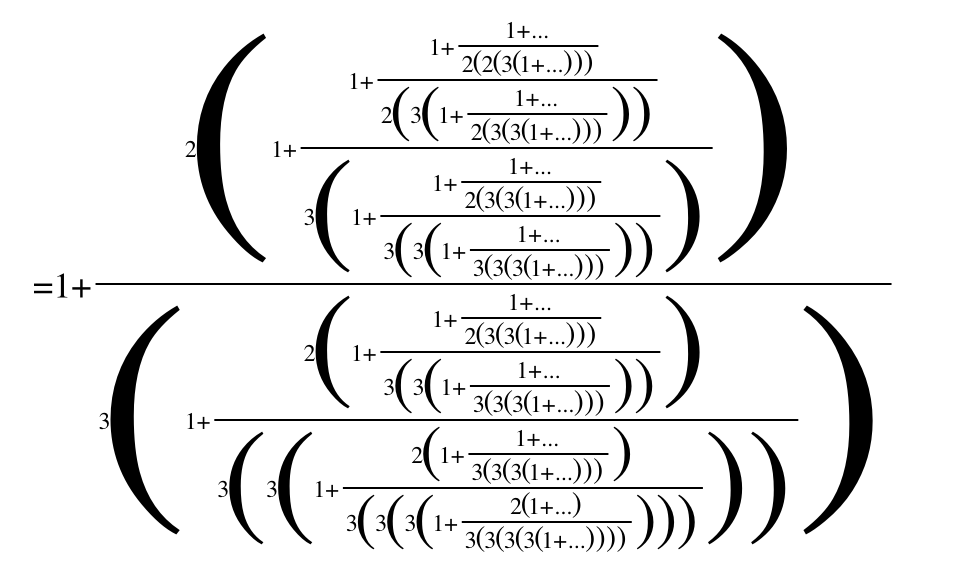# Worse than a continued fraction

Compute the result of the following:

$1 + \cfrac{2 + \cfrac{4 + \cfrac{8 + \ldots}{12 + \ldots}}{6 + \cfrac{12 + \ldots}{18 + \ldots}}}{3 + \cfrac{6 + \cfrac{12 + \ldots}{18 + \ldots}}{9 + \cfrac{18 + \ldots}{27 + \ldots}}}$

Informally, you have something like $f(x) = x + \frac{f(2x)}{f(3x)}$ for all $x$; expand $f(1)$ to infinity and compute its result.

(Note that I don't know the result; if I knew, I would have made this a problem. Source: friend's tweet)Note by Ivan Koswara
6 years ago

This discussion board is a place to discuss our Daily Challenges and the math and science related to those challenges. Explanations are more than just a solution — they should explain the steps and thinking strategies that you used to obtain the solution. Comments should further the discussion of math and science.

When posting on Brilliant:

• Use the emojis to react to an explanation, whether you're congratulating a job well done , or just really confused .
• Ask specific questions about the challenge or the steps in somebody's explanation. Well-posed questions can add a lot to the discussion, but posting "I don't understand!" doesn't help anyone.
• Try to contribute something new to the discussion, whether it is an extension, generalization or other idea related to the challenge.

MarkdownAppears as
*italics* or _italics_ italics
**bold** or __bold__ bold
- bulleted- list
• bulleted
• list
1. numbered2. list
1. numbered
2. list
Note: you must add a full line of space before and after lists for them to show up correctly
paragraph 1paragraph 2

paragraph 1

paragraph 2

[example link](https://brilliant.org)example link
> This is a quote
This is a quote
    # I indented these lines
# 4 spaces, and now they show
# up as a code block.

print "hello world"
# I indented these lines
# 4 spaces, and now they show
# up as a code block.

print "hello world"
MathAppears as
Remember to wrap math in $$ ... $$ or $ ... $ to ensure proper formatting.
2 \times 3 $2 \times 3$
2^{34} $2^{34}$
a_{i-1} $a_{i-1}$
\frac{2}{3} $\frac{2}{3}$
\sqrt{2} $\sqrt{2}$
\sum_{i=1}^3 $\sum_{i=1}^3$
\sin \theta $\sin \theta$
\boxed{123} $\boxed{123}$

Sort by:

Intuitively it would seem like $f(x)$ is an increasing function. Rearranging the recursive function gives $f(3x)(f(x)-x)=f(2x)$ so $|f(x)-x| < 1$. That would imply that $f(x)$ increases approximately linearly, but then again plugging in $f(x)=x+c$ gives no solution.

- 6 years ago

The solution to $c$ in $f(3x)(f(x)-x)=f(2x)$ where $f(x)=\sqrt[n]{x^n+c}$ as $n\to \infty$ and $x=1$ appears to be unbounded, so this path seems to be a dead end. Of course, all the math I'm doing is pretty hand-wavy to begin with.

- 6 years ago

An amazing idea !!

- 6 years ago

Some code to compute with different starting values and iteration depth: here

It's interesting to see that things converge quickly and that it is indeed approximately linear.

- 6 years ago

In fact, for large $x$, we have $f(x) \to x + \frac{2}{3}$ (as expected?).

- 6 years ago

Indeed. If we assume that $f(x)\sim x+c$ as $x\to\infty$, then $f(2x)/f(3x)\sim(2x+c)/(3x+c)\to2/3$ in the limit. Consistency then implies that $c=2/3$.

- 7 months, 2 weeks ago

The answer is definitely $\frac{5}{3}$. Here's as far as I've gotten in proving it:If you follow the fraction backward far enough you'll begin to realize that the terms inside the biggest parentheses tend towards $1$ as the fraction expands to infinity. This gives $1+\frac{2}{3}=\frac{5}{3}$, but this isn't exactly a proof, more a heuristic argument.

- 6 years ago

Well...

The answer is definitely $\frac{5}{3}$.

this isn't exactly a proof, more a heuristic argument.

I think they are quite contradictory.

I'm also pretty confident the answer is much closer to the one found by iteration (slightly less than $\sqrt{3}$) than $\frac{5}{3}$. Intuitively (not yet proven!), any of the fractions has value lying in $(0, 1)$. You can thus bound the result by replacing fractions to deep in the iteration with one of the two extreme values appropriately:

\begin{aligned} &1 + \cfrac{2 + \cfrac{4 + 0}{6 + 1}}{3 + \cfrac{6 + 1}{9 + 0}} = \frac{200}{119} \approx 1.6806722 \\ \le &1 + \cfrac{2 + \cfrac{4 + \ldots}{6 + \ldots}}{3 + \cfrac{6 + \ldots}{9 + \ldots}} \\ \le &1 + \cfrac{2 + \cfrac{4 + 1}{6 + 0}}{3 + \cfrac{6 + 0}{9 + 1}} = \frac{193}{108} \approx 1.7870370 \end{aligned}

- 6 years ago

This is definetly a mind bender

that's what I thought initially. However, if you track the fractions, you are not correctly removing the factors of 2 and 3.

Staff - 6 years ago

This problem really intrigues me, it seems so simple to state yet so hard to prove!

- 6 years ago